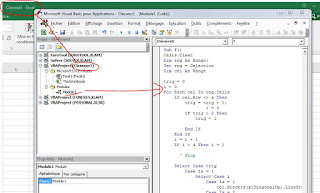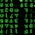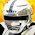## Friday, July 20, 2018

### Hexagonal Map Macro for Excel

How to make a hexagonal map very easily with Excel

Following recent discussions on A6 solo notebooks, the subject of hexagonal maps was addressed. Todd Zircher, whose excellent site I recommend http://www.tangent-zero.com/ gave me the tips to make a hexagonal map with Excel.

So I made a simple macro to draw hexagons. Simply select the area where you want the map to appear and launch the macro.Here's the code:

Sub HexMap()
Cells.Clear
Dim rng As Range:
Set rng = Selection
Dim cel As Range

trig = 0
i = 0

For Each cel In rng.Cells
If cel.Row <> a Then
trig = trig + 1:
i = 0
If trig > 2 Then
trig = 1
End If
End If

i = i + 1
If i > 4 Then i = 1

Select Case trig
Case Is = 1
Select Case i
Case Is = 1
cel.Borders(xlDiagonalUp).LineStyle = xlContinuous
Case Is = 2
cel.Borders(xlEdgeTop).LineStyle = xlContinuous
Case Is = 3
cel.Borders(xlDiagonalDown).LineStyle = xlContinuous
Case Is = 4
cel.Borders(xlEdgeBottom).LineStyle = xlContinuous
End Select
Case Is = 2
Select Case i
Case Is = 1
cel.Borders(xlDiagonalDown).LineStyle = xlContinuous
Case Is = 2
cel.Borders(xlEdgeBottom).LineStyle = xlContinuous
Case Is = 3
cel.Borders(xlDiagonalUp).LineStyle = xlContinuous
Case Is = 4
cel.Borders(xlEdgeTop).LineStyle = xlContinuous
End Select
End Select
a = cel.Row

Next cel
End Sub

For those who do not know vba, when you are in Excel:Then, you just have to select the area to draw and launch the macro (put the cursor anywhere inside the macro and press F5)

1.1.